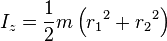# Moment of inertia (disk with off center hole)

#### Imperitor

I have a disk with some thickness to it and I need its moment of inertia.
So this is the formula with r1=0Now there is a "circular hole of diameter 'd' at a distance of 'r' from the geometric center of the disk." So I'm thinking that I should subtract the MoI of the hole from the disk. Here is a picture if you need it.

http://img99.imageshack.us/img99/782/50817033.jpg [Broken]

I could use the same MoI equation as I did for the disk on the hole but that doesn't account for the hole being off centered. Any ideas??

I need to eventually equate this to a small mass rotating around a center point, so if there is a more direct approach please tell me. Thanks in advance for the help.

Last edited by a moderator:

#### tiny-tim

Homework Helper
Welcome to PF!

Hi Imperitor ! Welcome to PF!I could use the same MoI equation as I did for the disk
That's right!And then use the parallel axis theorem to find the moment of inertia about an axis not through the centre of mass.#### Imperitor

Thanks. I really should have known that...

I'm really enjoying this forum. It's a great resource for an engineering student.

#### Imperitor

Wait a minute... hit another snag. To use that theorem I need to know what the mass of the missing hole is. I only know the mass of the disk as is (with the hole in it). Also the first formula I gave could only be used if I knew what the mass of the disk was with the hole filled in. This is driving me nuts. I can't even start working on the question I'm doing without getting this MoI.

#### tiny-tim

Homework Helper
just got up… :zzz:
Wait a minute... hit another snag. To use that theorem I need to know what the mass of the missing hole is. I only know the mass of the disk as is (with the hole in it). Also the first formula I gave could only be used if I knew what the mass of the disk was with the hole filled in. This is driving me nuts. I can't even start working on the question I'm doing without getting this MoI.
Easy-peasy …

divide the given mass by the area, to give you the densitythen multiply by each area separately!•harmyder

### The Physics Forums Way

We Value Quality
• Topics based on mainstream science
• Proper English grammar and spelling
We Value Civility
• Positive and compassionate attitudes
• Patience while debating
We Value Productivity
• Disciplined to remain on-topic
• Recognition of own weaknesses
• Solo and co-op problem solving